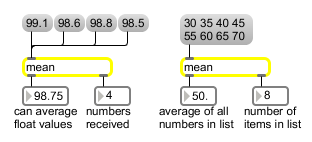# mean

Find the running average of a stream of numbers

## Description

mean calculates the mean (average) of all the numbers it has received and outputs it.

None.

## Messages

 bang Sends out the previous output (the stored average value). int input [int] The number is added to the sum of all numbers received up to that point, and the mean is sent out. float input [float] The number is added to the sum of all numbers received up to that point, and the mean is sent out. list input [list] The numbers in the list are added together, the sum is divided by the number of items in the list, and the mean is sent out. All previously received numbers are cleared from memory. clear Resets the contents of the object to zero.

## Output

float: Out left outlet: The mean (average) value of all numbers received up to that point, or of all the numbers received together in a list.

Out right outlet: How many numbers have been included in the averaging process.
int: Out left outlet: The mean (average) value of all numbers received up to that point, or of all the numbers received together in a list.

Out right outlet: How many numbers have been included in the averaging process.

## ExamplesFind the average value of many numbers